A certain loudspeaker system emits sound isotropically (uniformly in all directions) with a frequency of 1,054 Hz and an intensity of 6.429

Question

A certain loudspeaker system emits sound isotropically (uniformly in all directions) with a frequency of 1,054 Hz and an intensity of 6.429 mW/m2 at a distance of 7.945 m. Assume that there are no reflections. What is the intensity in 10-3 W/m2 at 39.3 m? (you don’t need to write the power/prefix and unit

in progress 0
6 months 2021-07-28T14:52:23+00:00 1 Answers 0 views 0

The intensity in 10-3 W/m2 at 39.3 m is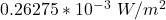Explanation:

Given Data:

frequency=1,054 Hz

intensity=6.429 mW/m^2

distance=7.945 m.

Required:

intensity in 10^-3 W/m^2 at 39.3 m=?

Solution:

Intensity at distance r from point source which is emitting sound waves of power P is given by the following formula:

Intensity=I=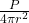Where:

P is the power

r is the distance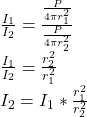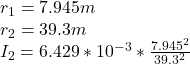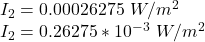The intensity in 10^-3 W/m2 at 39.3 m is